# How to Make and Read Back-to-Back Stem-and-Leaf Plots

How to Make and Read Back-to-Back Stem-and-Leaf PlotsBack-to-back stem-and-leaf diagrams show two sets of data on each side of the central stem.

Only the final digit of each number is listed in the leaf.

All of the preceding digits are listed in the stem.• The data for class 1 is written on the right of the stem and class 2 is written on the left of the stem.
• Only the last number is written in the leaves and the numbers get larger as we move away from the stem.
• In this case, the stem tells us the tens digit of the numbers in the leaves.
• The number in the stem tells us the tens digit for all of the numbers that follow in the leaf.
• The numbers in class 2 get larger as we go leftwards, away from the stem.

• The top row of data for class 2 is: 3, 6 and 8.
• The second row of data for class 2 is: 11, 12, 13, 14, 14, 17.
• The third row of data for class 2 is: 20 and 27.
• The fourth row of data for class 2 is: 32.

• The top row of data for class 1 is: 5 and 9.
• The second row of data for class 1 is: 10, 10, 12, 12, 15, 15, 16, 19.
• The third row of data for class 1 is: 23.
• The fourth row of data for class 1 is: 31.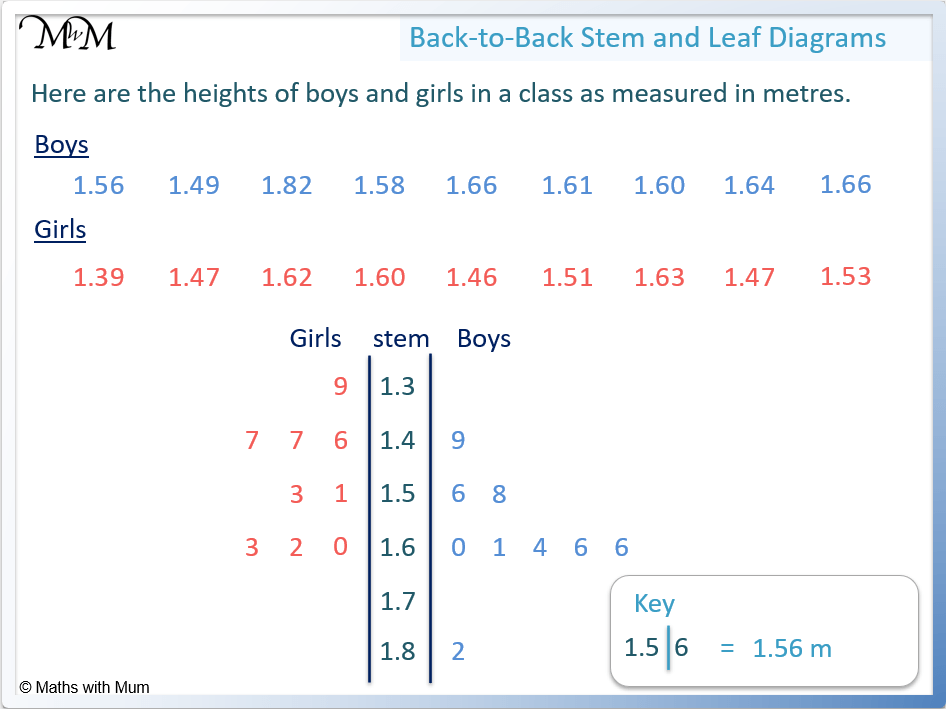• Only the final digit is listed in the leaves to represent each number.
• For example, in the girls data, 1.39 is listed by writing a 9 next to the 1.3 in the top row.
• In the second row, the girls have heights of 1.46, 1.47 and 1.46 listed. The boys have a height of 1.49.
• In the third row, the girls have heights of 1.51 and 1.53. The boys have heights of 1.56 and 1.58.

• In the fourth row, the girls have heights of 1.60, 1.62 and 1.62. The boys have heights of 1.60, 1.61, 1.64, 1.66 and 1.66.
• No girls or boys have any heights starting with 1.7.
• Finally, there is one boy who is 1.82 metres tall# Back-to-Back Stem-and-Leaf Plots

## What is a Back-to-Back Stem-and-Leaf Plot?

A back-to-back stem-and-leaf plot is used to compare two data distributions. The ‘stem’ is shared by both sets of data which are listed in the ‘leaves’ to the left and right of the stem respectively. The data is ordered so that the larger values are further from the stem.

The back-to-back stem-and-leaf plot below shows the data comparing the test scores of two classes.

In this example, the stem tells us the tens digit of each number and the numbers in the leaves tell us the ones digit.

For example, the 7 shown in line with the 2 in the stem represents 27.

The 5 shown in line with the 1 stem represents 15.On a back-to-back stem and leaf plot, a key is drawn to help explain how to read the diagram. The key on a stem-and-leaf plot illustrates an example.For example, 7 |1| means 17. The digit of 7 is in the left leaf and so, it is read backwards.

|1| 2 means 12. Since the 2 is in the right leaf, the number is read forwards.

## How to Draw a Back-to-Back Stem-and-Leaf Diagram

To make a back-to-back stem-and-leaf diagram:

1. Put the numbers in each data set in order from smallest to largest.
2. All apart from the final digit of each number is listed so that they appear in the central stem once.
3. The final digits in data set 1 are listed to the left of the stem, getting larger as they get further from the stem.
4. The final digits in data set 2 are listed to the right of the stem, getting larger as they get further from the stem.
1. For example, construct a back-to-back stem-and-leaf plot for the data shown. The stem and leaf plot shows the number of goals scored by each team over the season.

Team 1: 2, 9, 35, 21, 20, 10, 6, 13, 24, 31, 3, 1 Team 2: 5, 6, 19, 12, 15, 22, 30, 28, 21, 14, 17, 8

Step 1: Put the numbers in each data set in order from smallest to largest

Writing the numbers in order we obtain:

Team 1: 1, 2, 3, 6, 9, 10, 13, 20, 21, 24, 31, 35 Team 2: 5, 6, 8, 12, 14, 15, 17, 19, 21, 22, 28, 30Step 2: All apart from the final digit of each number is listed so that they appear in the central stem once

The digits listed in the stem will be the tens digits of each number. The ones digits of each number will go in the leaves.

The smallest value to list is a 1. It has zero tens and so, a 0 is written in the stem.

The largest values to list is a 35. It has 3 tens and so, the numbers in the stem will be listed from 0 up to 3.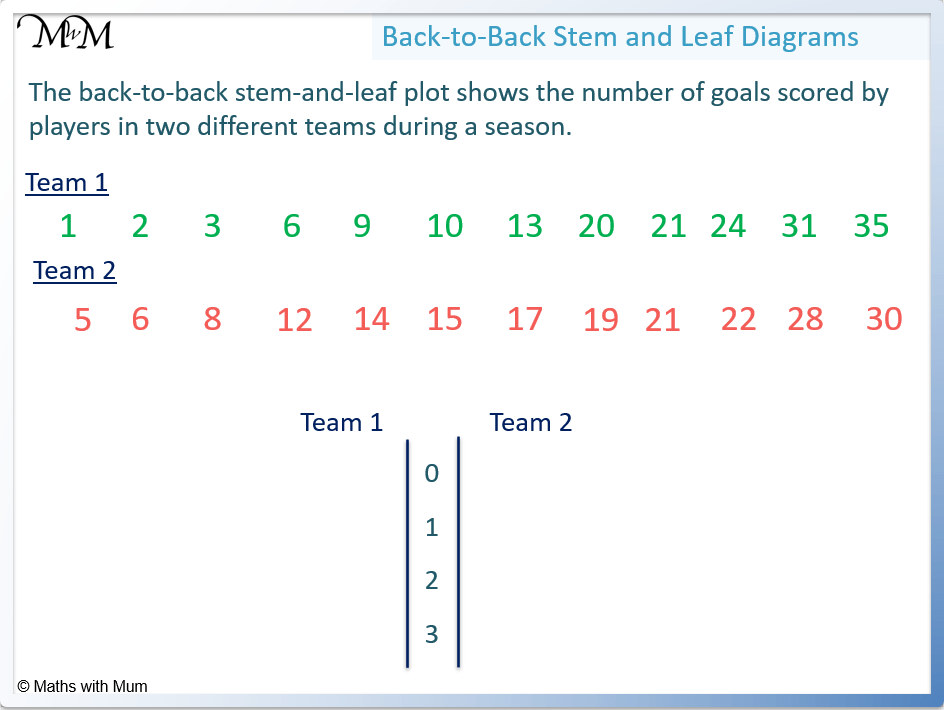Step 3: The final digits in data set 1 are listed to the left of the stem, getting larger as they get further from the stem

For example, entering the numbers with zero tens from team 1: we enter 1, 2, 3, 6 and 9.

These numbers are entered as 9 6 3 2 1 |0|.

The 0 in the stem shows that there is nothing in the tens column.

The numbers are listed backwards because they are listed so that the larger numbers are furthest from the stem.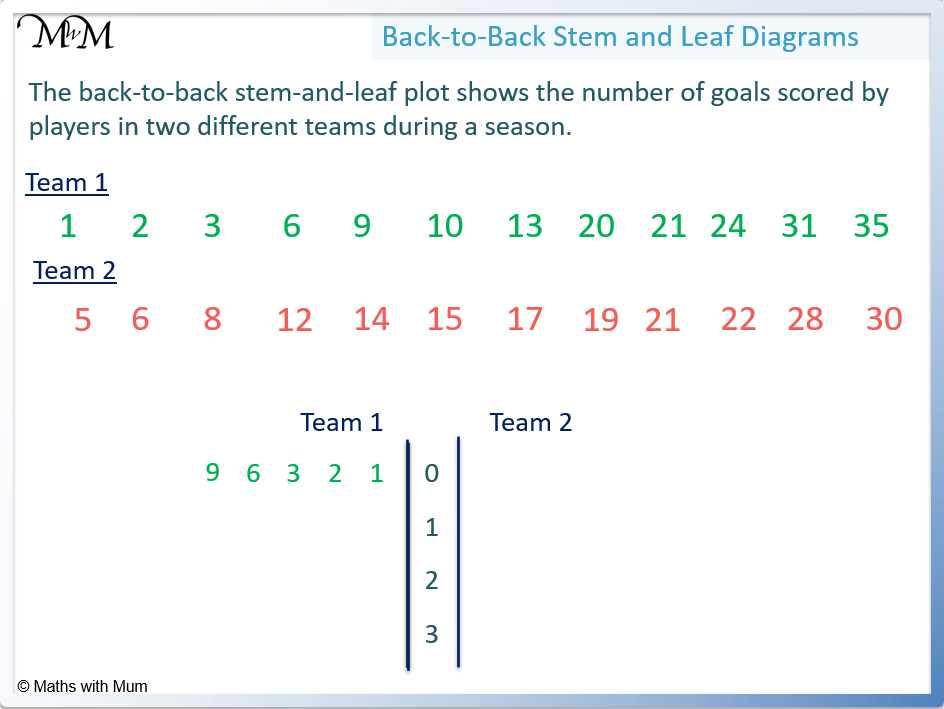The numbers of 10 and 13 are listed as 3 0 |1|.

Both numbers have a 1 in the tens column, so the stem has a 1 in it.

The numbers of 20, 21 and 24 are listed as 4 1 0 |2|.

The numbers of 31 and 35 are listed as 5 1 |3|.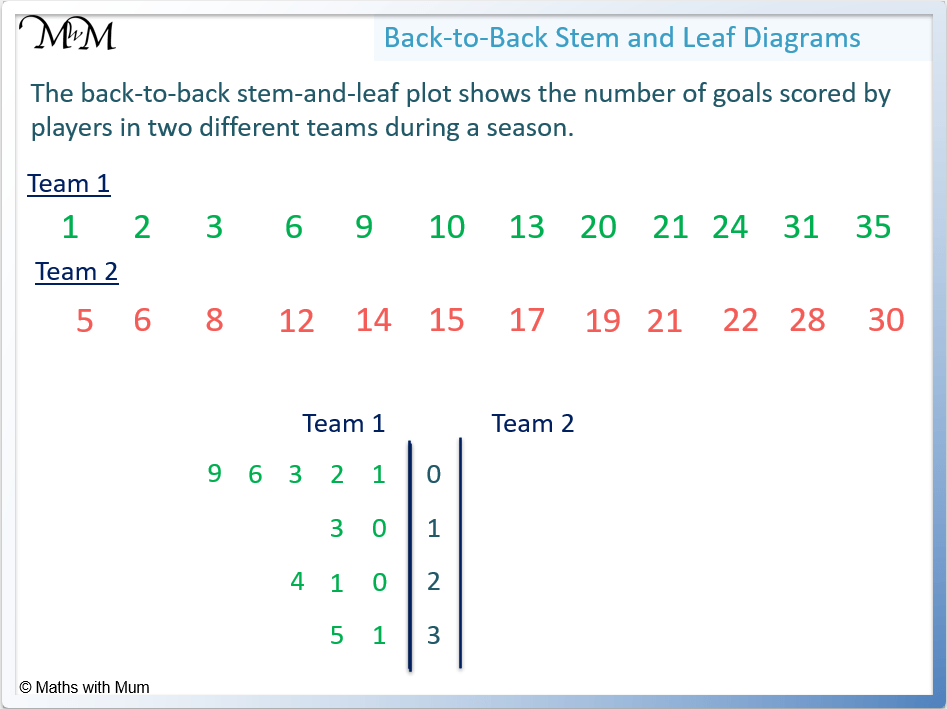Step 4: The final digits in data set 2 are listed to the right of the stem, getting larger as they get further from the stem

5, 6 and 8 are listed as |0| 5 6 8.

12, 14, 15, 17 and 19 are listed as |1| 2 4 5 7 9.

21, 22 and 28 are listed as |2| 1 2 8.

30 is listed as |3| 0.## Back-to-Back Stem-and-Leaf Plot with 3-Digit Numbers

When constructing a stem-and-leaf plot with 3-digit numbers, the hundreds and tens digits will go in the stem and the ones digit of each number will go in the leaves.

The back-to-back stem-and-lead plot shown below represents the heights (in centimetres) of two samples of plants.

Listing the values in sample 1:

101, 102, 102 and 108 are listed as 8 2 2 1 |10|.

This is because they all have a 1 and a 0 in their hundreds and tens columns respectively. Only the ones digit of each number is listed.

115 is listed as 5 |11|.

123, 125 and 129 are listed as 9 5 3 |12|.

134 and 137 are listed as 7 4 |13|.

140 and 145 are listed as 5 0 |14|.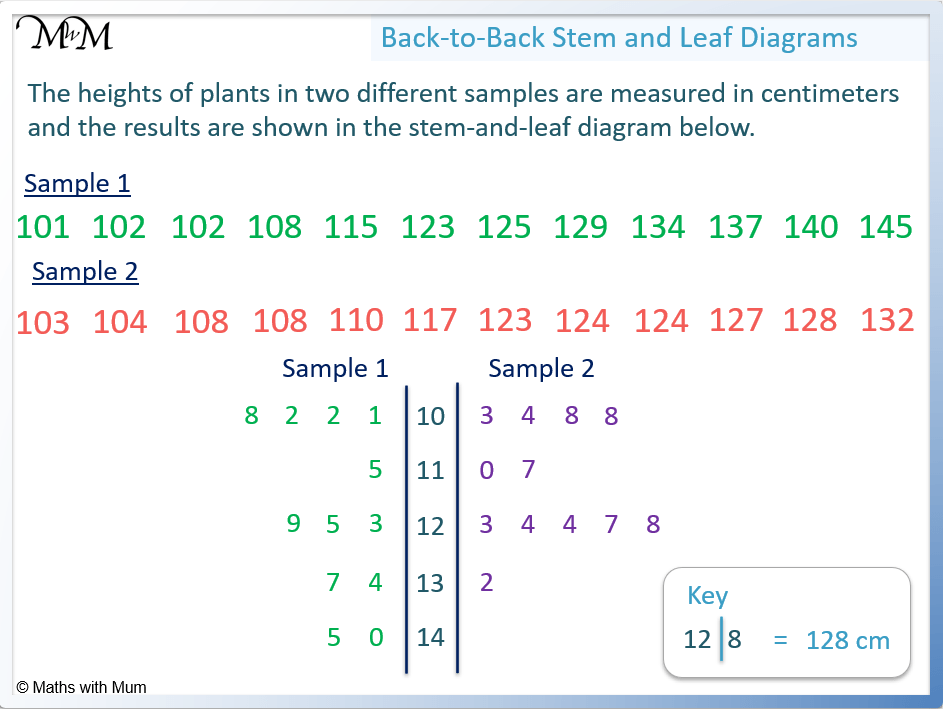Listing the values in sample 2:

103, 104, 108 and 108 are listed as |10| 3 4 8 8.

110 and 117 are listed as |11| 0 7.

123, 124, 124, 127 and 128 are listed as |12| 3 4 4 7 8.

132 is listed as |13| 2.

There are no plants in sample 2 with a 1 in the hundreds column and a 4 in the tens column.

## Back-to-Back Stem-and-Leaf Plot with Decimals

Only the final decimal digit is listed in a stem-and-leaf plot. With decimal numbers, all other preceding digits are listed in the stem. The decimal point may also be listed in the stem.

For example, the following stem-and-leaf plot displays the heights of boys and girls in a class.

Listing the boy’s data:

1.49 is listed as |1.4| 9.

1.56 and 1.58 are listed as |1.5| 6 8.

1.60, 1.61, 1.64, 1.66 and 1.66 are listed as |1.6| 0 1 4 6 6.

1.82 is listed as |1.8| 2.Listing the girl’s data:

1.39 is listed as 9 |1.3|.

1.46, 1.47 and 1.47 are listed as 7 7 6 |1.4|.

1.51 and 1.53 are listed as 3 1 |1.5|.

1.60, 1.62 and 1.63 are listed as 3 2 0 |1.6|.

## How to Use a Back-to-Back Stem-and-Lead Plot to Compare Data Sets

Back-to-back stem-and-leaf plots are used to compare two data sets. They are a visual display of the shape of the data, so that skewness, range and modal values can more easily be seen. The sizes of the values within the two data sets can easily be compared by looking at their general position on the diagram.

For example, the back-to-back stem-and-leaf plot below shows the heights of boys and girls in a class. The mode, median, range and skewness of the data can easily be found from the diagram.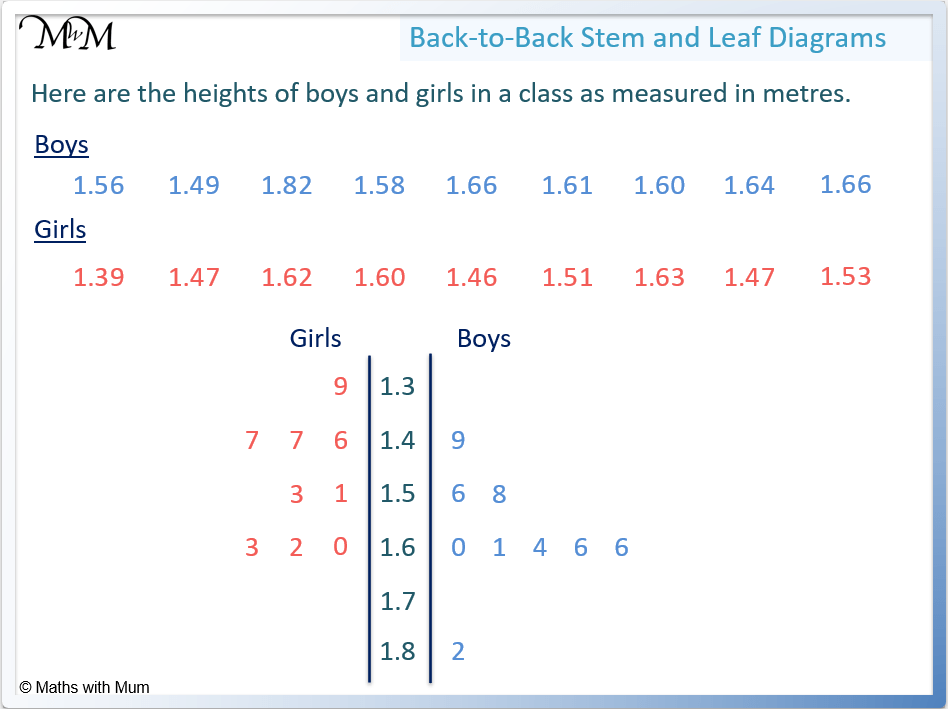The mode from a stem-and-leaf plot is found by looking for the digit that is repeated the most in a particular row.

The mode for the girl’s data is seen to be 1.47 m. This is because the only digit that is repeated in this data set is a 7 in the |1.4| stem.

The mode for the boy’s data is 1.66 m. This is because the only digit that is repeated in this data set is a 6 in the |1.6| stem.

The mode is a type of average and since the boys have a larger mode, we can interpret this as the boys having a larger height on average.

The median is found in the middle of each data set on a stem-and-leaf plot.

There are 9 data values in each set of data. By crossing off the smallest 4 data values and the largest 4 data values, the middle value will be left. This is the median.

In the girl’s data, the values of 1.39, 1.46, 1.47 and 1.47 are crossed off at the bottom, along with 1.63, 1.62, 1.60 and 1.53 at the top.

This leaves 1.51 metres as the median height for the girls.

In the boy’s data, the values of 1.49, 1.56, 1.58 and 1.60 are crossed off at the bottom, along with 1.82, 1.66, 1.66 and 1.64 at the top.

This leaves 1.61 metres as the median height for boys.The median is a type of average and so, we can say that the boys are taller on average.

The range is found on a stem-and-lead plot by subtracting the first value from the last value

The first value in the girl’s data is 1.39 m. The last value is 1.63 m.

The range of the girl’s data is 1.63 – 1.39 = 0.24 m.

The first value in the boy’s data is 1.49 m. The last value is 1.82 m.

The range of the boy’s data is 1.82 – 1.49 = 0.33.

The range describes the spread of the data. Since the boys have a larger range, the boy’s heights are more spread than the girls.

The skewness of the data on a stem-and-leaf plot can be found by drawing around the peaks of each row.

The skew of the data can be seen below.

The girl’s data forms two peaks and therefore we can call it bimodal.

The boy’s data starts with a slow climb and most of the data is bunched at the bottom. This is called negatively skewed data.

In the opposite case, where most of the data is bunched at the top, this would be called positively skewed data.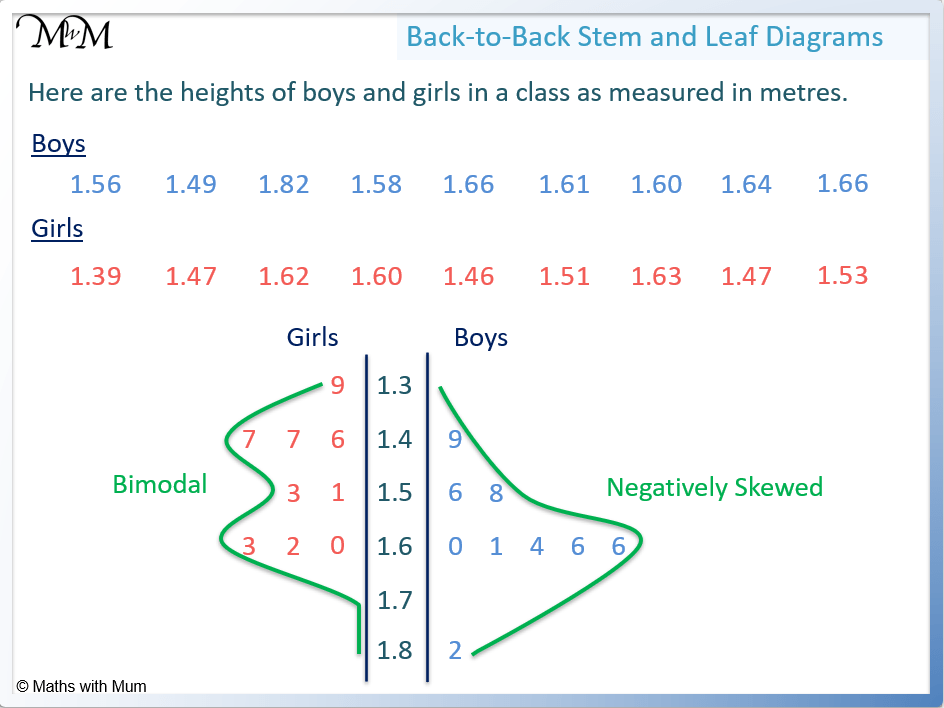A stem-and-leaf plot easily shows the positions of the data values.

It is easy to see that the boy’s values are typically lower down in the diagram than the girl’s values.

This tells us that the boy’s values are typically found in the larger numbers on the diagram and so, it is easy to see that boys are taller than girls on average in this example.Now try our lesson on Probability with Spinners where we learn how to understand probability with spinners.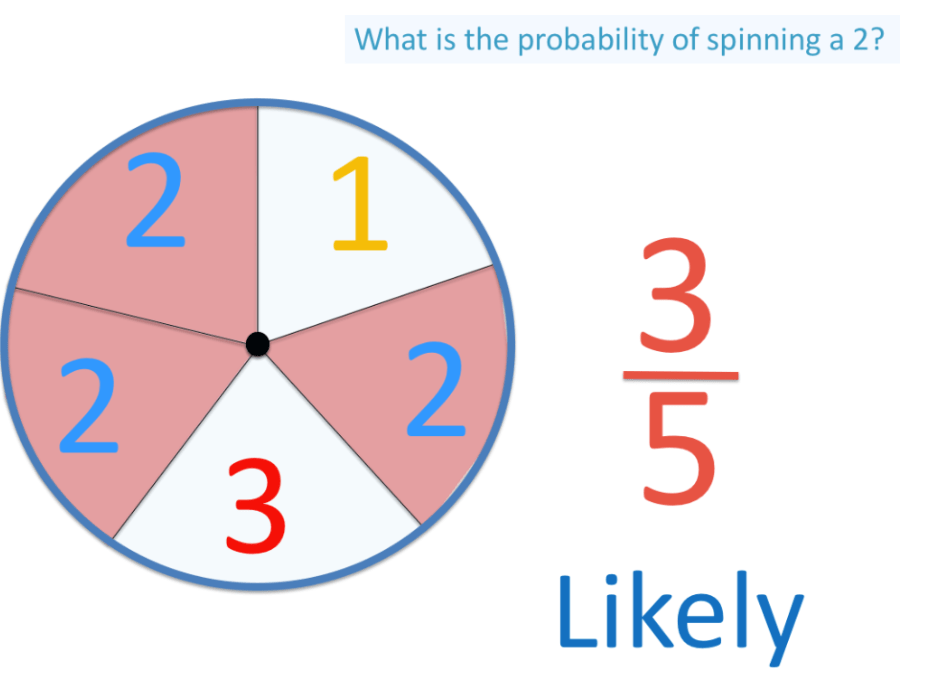error: Content is protected !!